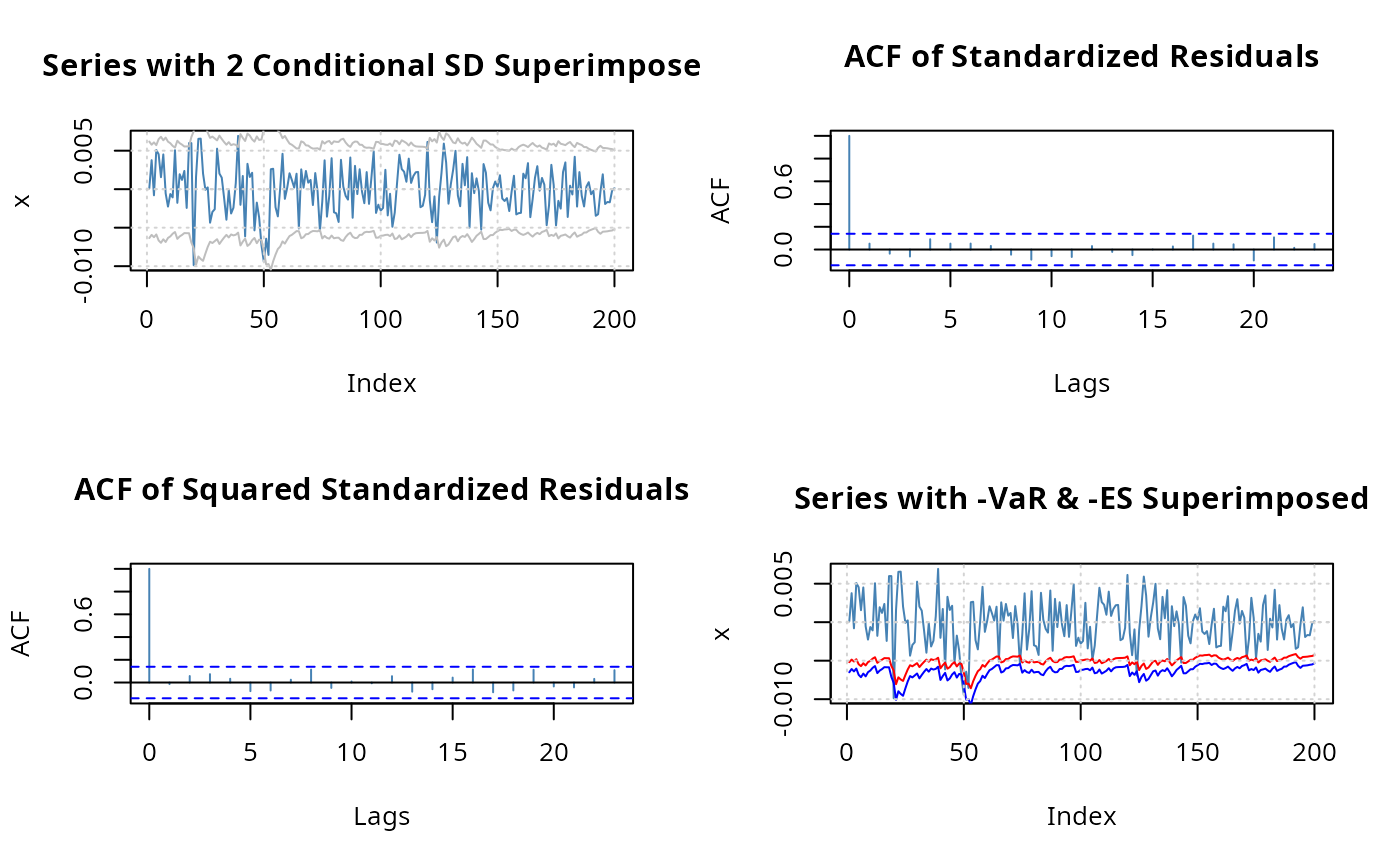Plot methods for GARCH Modelling.

## Usage

# S4 method for fGARCH,missing
plot(x, which = "ask", ...)

## Arguments

x

an object of class "fGARCH".

which

a character string or a vector of positive denoting which plot should be displayed, see section ‘Details’.

...

optional arguments to be passed.

## Details

The plot method for "fGARCH" objects offers a selection of diagnostic, exploratory, and presentation plots from a menu. Argument which can be used to request specific plots, which is particularly useful in scripts.

The generic function plot allows to display 13 graphs:

 Time SeriesPlot Conditional Standard Deviation Plot Series Plot with 2 Conditional SD Superimposed Autocorrelation function Plot of Observations Autocorrelation function Plot of Squared Observations Cross Correlation Plot Residuals Plot Conditional Standard Deviations Plot Standardized Residuals Plot ACF Plot of Standardized Residuals ACF Plot of Squared Standardized Residuals Cross Correlation Plot between $r^2$ and r Quantile-Quantile Plot of Standardized Residuals

## Author

Diethelm Wuertz for the Rmetrics R-port

garchFit, class fGARCH,

predict, fitted, residuals

plot

## Examples

## garchSim -
# Default Garch(1,1) Model:
x = garchSim(n = 200)
#> GMT
#>                   garch
#> 2022-11-18  0.007522375
#> 2022-11-19 -0.001628817
#> 2022-11-20  0.003737262
#> 2022-11-21  0.004705047
#> 2022-11-22  0.001966999
#> 2022-11-23 -0.005850769

## garchFit -
fit = garchFit(formula = ~ garch(1, 1), data = x, trace = FALSE)

## Batch Plot:
plot(fit, which = 3)if (FALSE) {
## Plot:
# Interactive Plot:
plot(fit)
}